## Law of Sines

You can use the Law of Cosines discussed in the last section to solve general triangles, but only under certain conditions. The formulas that will be developed in this section provide more flexibility in solving these general triangles.

The following discussion centers around Figure 1 .Figure 1
Reference triangles for Law of Sines.

Line segment CD is the altitude in each figure. Therefore Δ ACD and Δ BCD are right triangles. Thus,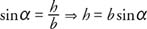In Figure (b), ∠CBD has the same measure as the reference angle for β Thus,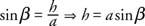It follows that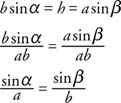Similarly, if an altitude is drawn from A,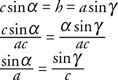Combining the preceding two results yields what is known as the Law of Sines.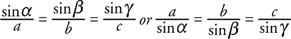In other words, in any given triangle, the ratio of the length of a side and the sine of the angle opposite that side is a constant. The Law of Sines is valid for obtuse triangles as well as acute and right triangles, because the value of the sine is positive in both the first and second quadrant—that is, for angles less than 180°. You can use this relationship to solve triangles given the length of a side and the measure of two angles, or given the lengths of two sides and one opposite angle. (Remember that the Law of Cosines is used to solve triangles given other configurations of known sides and angles).

First, consider using the Law of Sines to solve a triangle given two angles and one side.

Example 1: Solve the triangle in Figure  given θ = 32°, χ = 77°, and d = 12.Figure 2
Drawing for Example 1.

It follows from the fact that there are 180° in any triangle that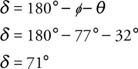From the Law of Sines, the following relationships are obtained:Solving as two independent proportions,Example 2: Solve the triangle in Figure given α = 125° β =35°, and b, = 42.Figure 3
Drawing for Example 2.

From the fact that there are 180° in any triangle, then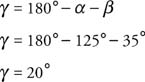Again, using the Law of Sines,The second use of the Law of Sines is for solving a triangle given the lengths of two sides and the measure of the angle opposite one of them. In this case, it is possible that more than one solution will exist, depending on the values of the given parts of the triangle. The next example illustrates just such a case.

Example 3: Solve the triangle(s) given a = 13,1, = 20, and a = 35°.Figure 4
Drawing for Example 3.

Figure shows two possible positions for side a. This will occur if a > b To determine if a is greater than h, the value of h is found.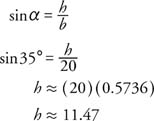Therefore, a > h and the diagram shows two solutions for the triangle. From the Law of Sines,Because sin β is positive in both quadrant I and quadrant II, β can have two values and therefore two different solutions for the triangle.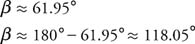Solution 1: The sum of the angles of a triangle is 180°. Thus,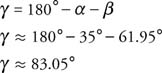Using the Law of Sines,Solution 2: The sum of the angles of a triangle is 180°. Thus,Using the Law of Sines,